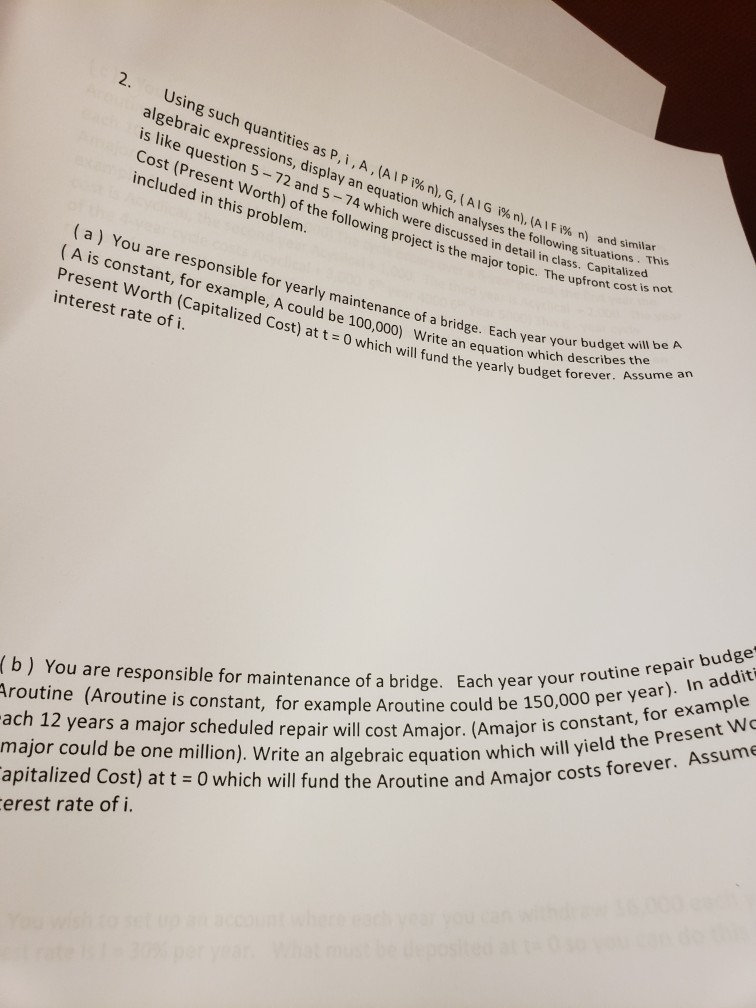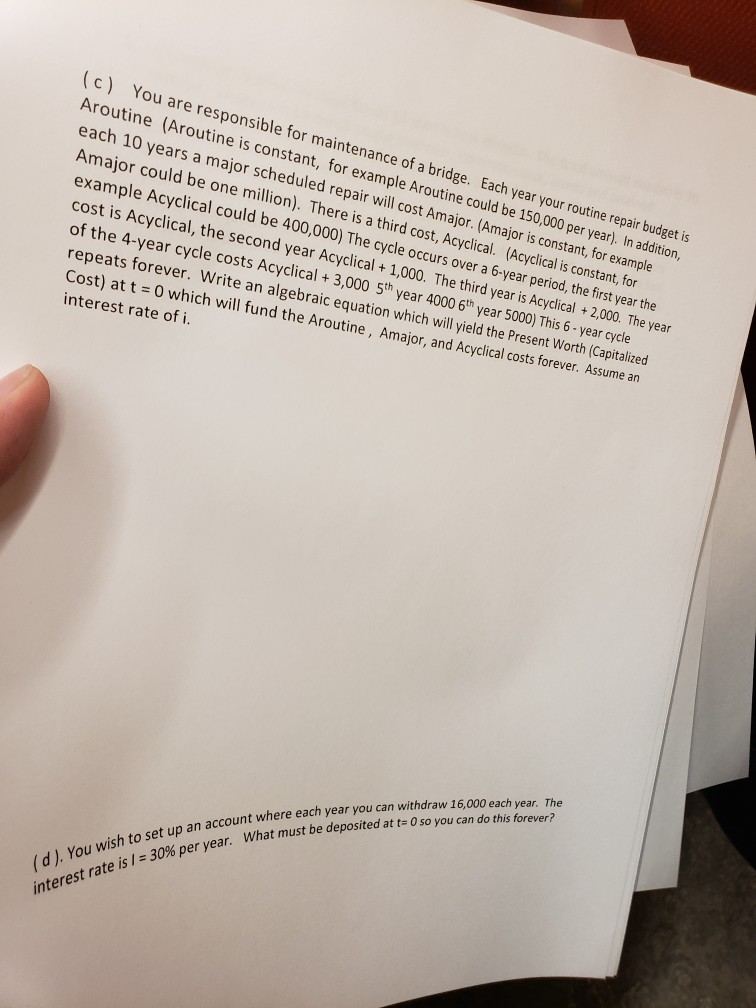# 2. Using such quantities as P, i , A , (A I P i% n), G, ( Al G i%n), (AIF î% n) algebraic express...2. Using such quantities as P, i , A , (A I P i% n), G, ( Al G i%n), (AIF î% n) algebraic expressions, display an equation which analy is like question 5-72 Cost (Present Worth) of the following project is the major topic. The uptror included in this problem. and 5-74 which were discussed in detail in class. Capitalize n) and similar ses the e following situations. This (a) You are responsible for yearly maintenance of a bridge. Eacn veanich de (A is constant, for example, A could be 100 Each year your budget will be A nt Worth (Capitalized Cost) at t 0 which will fund the yearly budget foree rite an equation which describes the interest rate of i. Each year your 150,000 per year). In addit routine (Aroutine is constant, for example Aroutine could be l ach 12 years a major scheduled repair will cost Amajor. (Amajor is ie major could be one million). Write an algebraic equation which apitalized Cost) at t O which will fund the Aroutine and Amajor costs fo erest rate of i. (b) You are responsible for maintenance of a bridge. will yield the Present Wo
(c) You are responsible for maintenance of a bridge. Each year your routine repair budget is Aroutine (Aroutine is constant, for example Aroutine could be 150,000 per year). In addition, each 10 years a major scheduled repair will cost Amajor Amajor could be one million). There is a thi example Acyclic cost is Acyclical, the second year Acyclical + 1,000. The third yea is Acydical of the 4-year cycle costs Acyclical 3,000 sth year 4000 6h year 500 This 6- year cycde repeats forever. Write an algebraic equation which will yield the Present Worth (Capitalized Cost) at t 0 which will fund the Aroutine, Amajor, and Acyclical costs forever. Assume an interest rate of i. . (Amajor is constant, for example rd cost, Acyclical. (Acyclical is constant, for al could be 400,000) The cycle occurs over a 6-year period, the first year +2,000 The year year. The year (d J. You wish to an account interest rate is l 30% eeach , set up an account where each year you can withdraw 16,000 each What must be deposited at t 0 so you can do this for What m per year.

a). If annual amount = A, interest rate = i and n = infinity then capitalized cost is

A*(P/A, i, n)

Note: For n = infinity, (P/A, i, n) will be reduced to 1/i

b). Repair cost/year = Aroutine; major repair every 12 years = Amajor

Convert Amajor to an annulized cost first: Amajor*(A/F, i, 12)

Convert this annualized cost into the PW: Amajor*(A/F, i, 12)*(P/A, i, n)

Capitalized cost = Aroutine*(P/A, i, n) + Amajor*(A/F, i, 12)*(P/A, i, n)

c). Repair cost/year = Aroutine; major repair every 10 years = Amajor; cyclical cost for every 6 years = Acyclical increasing by 1,000 per year

Step 1). Calculate the PW of Acyclical arithmetic gradient at the beginning of year 1 (or beginning of year 7, etc.)

Gradient (G) = 1,000 (amount by which Acyclical is increasing every year)

PW of Acyclical (PW1) = Acyclical*((P/A, i, 6) + G*(P/G, i, 6)

Step 2). Now, this PW1 will be repeating every 5 years (beginning of year 6), so its annualized cost over a 5 year period will be PW1*(A/F, i, 5)

Step 3). Covert this to the capitalized cost as PW1*(A/F, i, 5)*(P/A, i, n)

Step 4). PW of Amajor = Amajor*(A/F, i, 120)*(P/A, i, n)

Step 5). PW of Aroutine = Aroutine*(P/A, i, n)

Step 6). Total capitalized cost = step 5 + step 4 + step 3

= Aroutine*(P/A, i, n) + Amajor*(A/F, i, 120)*(P/A, i, n) + PW1*(A/F, i, 5)*(P/A, i, n) OR

Aroutine*(P/A, i, n) + Amajor*(A/F, i, 120)*(P/A, i, n) + [Acyclical*((P/A, i, 6) + G*(P/G, i, 6)]*(A/F, i, 5)*(P/A, i, n)

d). Perpetuity amount = Annual withdrawal/annual interest rate = 16,000/30% = 53,333.33

##### Add Answer of: 2. Using such quantities as P, i , A , (A I P i% n), G, ( Al G i%n), (AIF î% n) algebraic express...
More Homework Help Questions Additional questions in this topic.